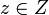Center is local powering-invariant

This article gives the statement, and possibly proof, of the fact that for any group, the subgroup obtained by applying a given subgroup-defining function (i.e., center) always satisfies a particular subgroup property (i.e., local powering-invariant subgroup)}
View subgroup property satisfactions for subgroup-defining functions$|$ View subgroup property dissatisfactions for subgroup-defining functions

Statement

The center of a group is a local powering-invariant subgroup. Explicitly, suppose$G$ is a group and$Z$ is the center. Suppose$z \in Z$ and$n$ is a natural number such that there is a unique$x \in G$ satisfying$x^n = z$. Then,$x \in Z$.

Facts used

1. Group acts as automorphisms by conjugation

Proof

Given: Group$G$ with center$Z$. Element$z \in Z$ and natural number$n$ such that there exists a unique$x \in G$ satisfying$x^n = z$.

To prove:$x \in Z$. In other words,$yxy^{-1} = x$ for all$y \in G$.

Proof: We have by Fact (1) that:$\! (yxy^{-1})^n = yx^ny^{-1}$

Simplifying further, we get that:$\! (yxy^{-1})^n = yx^ny^{-1} = yzy^{-1} = z$

where we use that$x^n = z \in Z$. Since$x$ is the unique element of$G$ whose <mah>n^{th}[/itex] power is$z$, the above forces that$yxy^{-1} = x$.